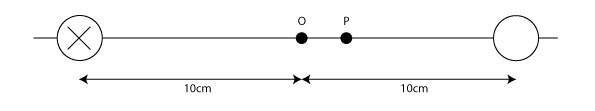# Magnetic fields and the right hand grip rule

## Homework Statement

(i) Find the magnitude and direction of the magnetic field at the point O located at a distance D = 10 cm from a wire carrying a current i = 1.0 A directed into the page, as shown in the attached drawing.

(ii) A second wire, identical to the first, is placed a distance D = 10 cm on the opposite side of O from the first wire as shown in Figure 3. This second wire carries a current with the same magnitude of i = 1.0 A. In which direction must this current flow in order for the magnetic field strength at O to be non* zero?

## Homework Equations

Right hand grip rule
B(r) = μ i / 2π r

## The Attempt at a Solution

For (i) I've got the magnitude of the field as 2uT from the B(r) = u i / 2π r and the direction as downwards using the right hand grip rule.

For (ii) I'm assuming that for it to be non zero then the current would have to be directed out of the page - if it was into the page then the resultant would be zero?

#### Attachments

•Current-in-a-field.jpg
3.6 KB · Views: 710
Last edited:

SammyS
Staff Emeritus
Homework Helper
Gold Member

## Homework Statement

(i) Find the magnitude and direction of the magnetic field at the point O located at a distance D = 10 cm from a wire carrying a current i = 1.0 A directed into the page, as shown in the attached drawing.

(ii) A second wire, identical to the first, is placed a distance D = 10 cm on the opposite side of O from the first wire as shown in Figure 3. This second wire carries a current with the same magnitude of i = 1.0 A. In which direction must this current flow in order for the magnetic field strength at O to be non* zero?

## Homework Equations

Right hand grip rule
B(r) = u i / 2π r

## The Attempt at a Solution

For (i) I've got the magnitude of the field as 2uT from the B(r) = u i / 2π r and the direction as downwards using the right hand grip rule.

For (ii) I'm assuming that for it to be non zero then the current would have to be directed out of the page - if it was into the page then the resultant would be zero?(i) I didn't check the numbers, but that's the way to calculate the B-field at point O

For (ii), You have the correct result, but it's not so much an assumption as it is a well-reasoned conclusion.

Thank you SammyS (i) that's exactly what the question asked for and (ii) I thought so... cheers - I feel so much better now.